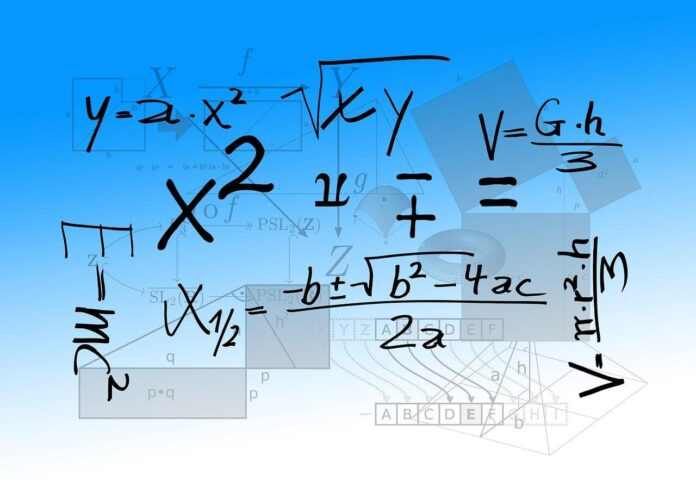# Students What they Need To Know About BODMAS?

562Mathematics is one of the most important subjects in the world and it will continue to be one forever. Nothing much can be done in today’s time without the influence of maths, isn’t it? Mathematics consists of hundreds of different formulas, equations, elements and what not. One such rule of maths is popularly known as Bodmas rule. You must have already heard or read about it somewhere! We are already accustomed with the basics of Mathematics which include addition, division, subtraction and multiplication. At a very early age, we are introduced to all of these methods.

But, what do we do when we face a problem that requires all of the above to be solved? Simple. We take the help of Bodmas rule. Bodmas has a huge application in the world of Mathematics and we can solve a whole lot of problems with it’s help. Keep reading our blog to know about how Bodmas is important and how it can help us in the long run.

## What Is The Bodmas Rule?

Bodmas is an acronym for Bracket, Of, Division, Multiplication, Addition and Subtraction. In many places, PEMDAS or (Parentheses, Exponents, Multiplication, Division, Addition and Subtraction) is the synonym for Bodmas. It helps to explain the order of the operations in order to solve any expression. As per the rule of Bodmas, if any expression contains brackets which can be any of the three – (), {}, [], we need to solve or simplify the bracket first.The acronyms are explained below:

The full form of Bodmas stands for –

• Brackets (Parts of a calculation inside the bracket always come first).
• Orders (powers and square roots)
• Division
• Multiplication
• Subtraction

Then it will be followed by powers and roots, etc. After that, the methods of division, multiplication, addition and subtraction will be commenced from the left to right. Keep in mind that, any problem solved in the wrong  order will only result in a wrong answer. Solving the problem in a systematic way is the true way by which you can solve it properly.

Also Read : Why a Nursery School Franchise is the Perfect Business Proposition for You?

## Bodmas Conditions and Rules

Bodmas comes with a set of conditions and rules which you need to follow properly. Without knowing them, you cannot solve any problem related to it properly. Take a look –

• x + (y + z) = x + y – z : Open the bracket and add all the available terms.
• x – (y + z) = x – y – z : Open the bracket and you need to multiply the negative sign with each of the term present inside the bracket. (All the positive terms will be negative and vice versa in the other case)
• x(y + z) = xy + xz : Multiply all the terms outside with each of the term inside the bracket.

To solve problems relating to Bodmas rule, one needs to carefully remember and make use of these rules and implement them. Any mistake while following these rules, will result in the overall mistake of the arithmetical problem.

## Some More Details Regarding Bodmas Rule

Here are some more important details that you should be well aware of regarding Bodmas –

Numbers – a number is a mathematical value that is used to represent a quantity and is used in the process of counting and making calculations. The symbol which represents the number is known as numeral. According to their special traits, numbers can be –

• Natural Numbers
• Integers
• Rational Numbers
• Irrational Numbers
• Real Numbers
• Complex Numbers
• Imaginary Numbers

Operators or operations: an operator is a character that is the combination of two numbers and produce any equation or expression. The most commonly used operators in the field of mathematics are –

• Addition : “ + “
• Subtraction: “ – “
• Multiplication: “ x “
• Division: “ ÷ ”

These Bodmas important questions with rules and methods you need to keep in mind while solving Bodmas rule problems. Without the use of these, you cannot simply solve problems of such kind.

## Conclusion

Bodmas is a very important rule in Mathematics. It helps us to solve big and complicated problems without much hassle. Hence, it is imperative to know every aspect of Bodmas if you want to solve Mathematical problems of higher levels later on. In the long run, Bodmas is a great technique which is going to benefit you in solving different issues.